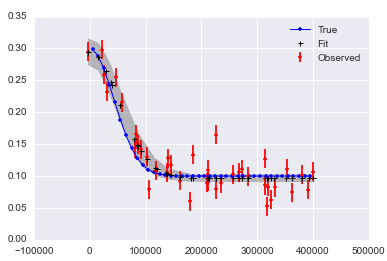# Monthly Archives: July 2014

## MCMC in Python: sim and fit with same model

Here is a github issue and solution that I saw the other day. I think it’s a nice pattern.

```def generate_model(values={'mu': true_param, 'm': None}):

#prior
mu = pymc.Uniform("mu", lower=-10, upper=10, value=values['mu'],
observed=(values['mu'] is not None))

# likelihood function
m = pymc.Normal("m", mu=mu, tau=tau, value=values['m'],
observed=(values['m'] is not None))

return locals()
```

Comments Off on MCMC in Python: sim and fit with same model

Filed under statistics

## MCMC in Python: Fit a non-linear function with PyMC

Here is a recent q&a on stack overflow that I did and liked.Comments Off on MCMC in Python: Fit a non-linear function with PyMC

Filed under statistics

## Tabular Data in Python: Getting just the columns I want from pandas.DataFrame.describe

The Python Pandas DataFrame object has become the mainstay of my data manipulation work over the last two years. One thing that I like about it is the `.describe()` method, that computes lots of interesting things about columns of a table. I often want those results stratified, and `.groupby(col)` + `.describe()` is a powerful combination for doing that.

*But* today, and many days, I don’t want all of the things that `.describe()` describes. And the ones that I do want, I want as columns. Here is the recipe for that:

```import pandas as pd

df = pd.DataFrame({'A': [0,0,0,0,1,1],
'B': [1,2,3,4,5,6],
'C': [8,9,10,11,12,13]})

df.groupby('A').describe().unstack()\
.loc[:,(slice(None),['count','mean']),]
```

and out comes just what I wanted:

```       B            C
count  mean  count  mean
A
0      4   2.5      4   9.5
1      2   5.5      2  12.5

```

It took me a while to figure this out, and these docs helped:
http://pandas.pydata.org/pandas-docs/stable/reshaping.html#reshaping-by-stacking-and-unstacking
http://pandas.pydata.org/pandas-docs/stable/indexing.html#indexing-xs

(Note: this requires Pandas version at least 0.14.)

Comments Off on Tabular Data in Python: Getting just the columns I want from pandas.DataFrame.describe

Filed under software engineering

## The one before that

Jake Vanderplas’s comparison of Python MCMC modules was preceded by a Bayesian polemic. In general, I find the stats philosophy war old-timey and distracting, but his comparison of confidence intervals and credible intervals is something I need to understand better.

http://jakevdp.github.io/blog/2014/06/12/frequentism-and-bayesianism-3-confidence-credibility/

Comments Off on The one before that

Filed under statistics